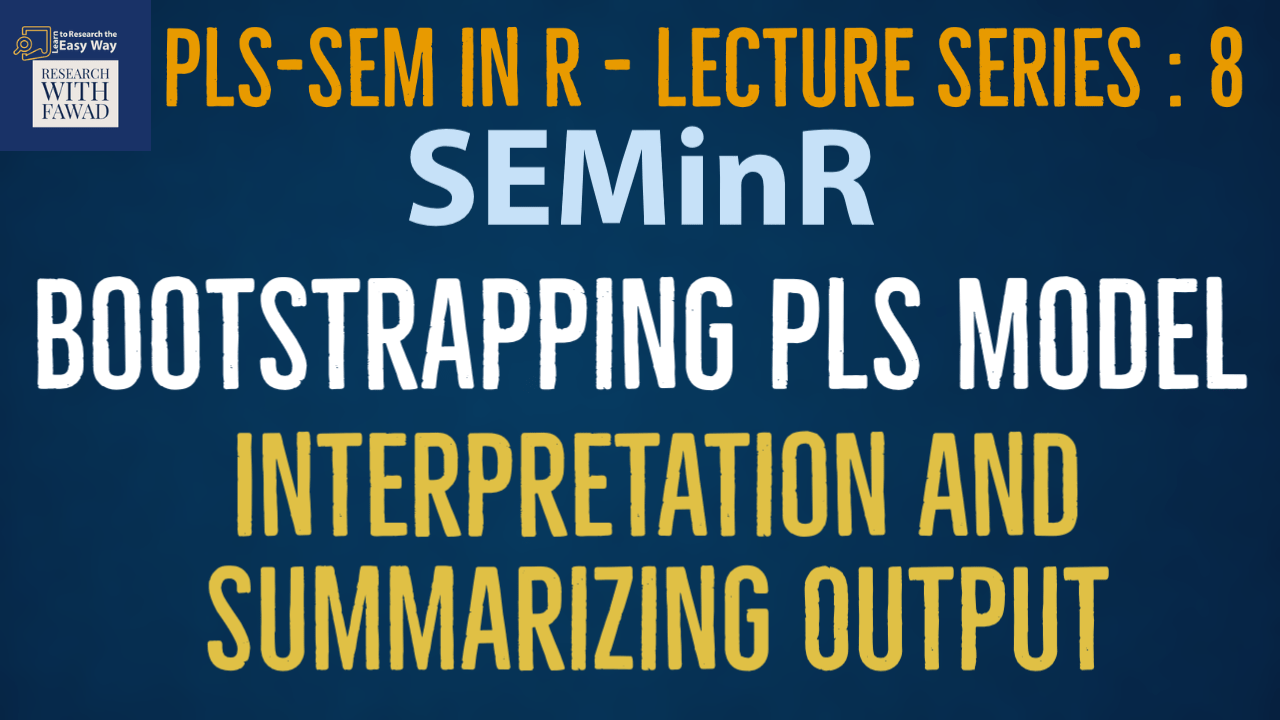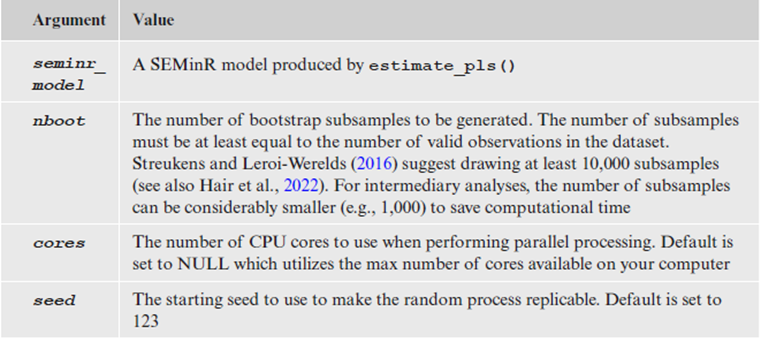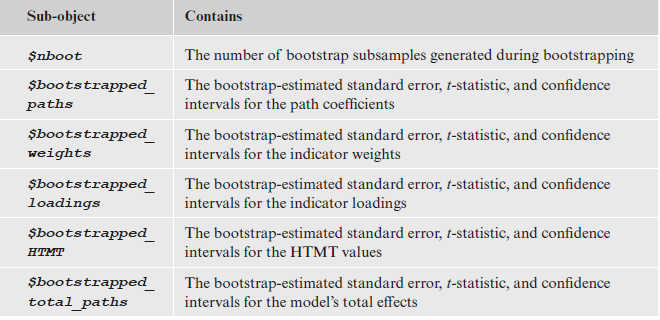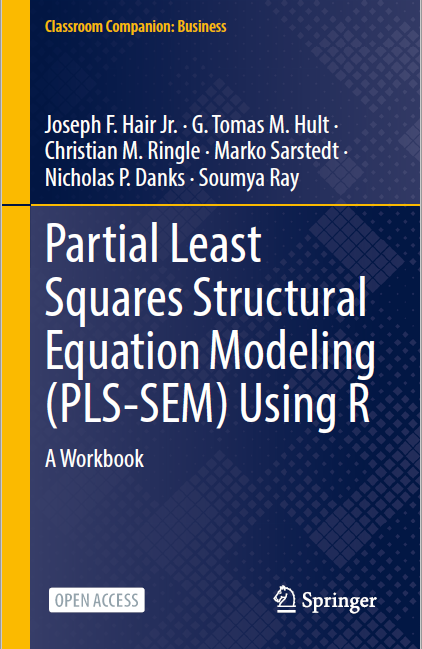# SEMinR Lecture Series Bootstrapping PLS Model### SEMinR Lecture SeriesBootstrapping PLS Model

This series of lecture on SEMinR Package will introduce the SEMinR package.

## SEMinR

There are four steps to specify and estimate a structural equation model using SEMinR:

• Specifying the measurement models
• Specifying the structural model
• Estimating, bootstrapping, and summarizing the model

## Bootstrapping

• PLS-SEM is a nonparametric method – thus, we need to perform bootstrapping to estimate standard errors and compute confidence intervals.
• The bootstrap_model() function is used to bootstrap a previously estimated SEMinR model (simple_model). The previously estimated pls model (the object holding the pls estimation is bootstrapped)
• This function applies the arguments shown in Table. In the example, we use the bootstrap_model() function and specify the arguments seminr_model = simple_model, nboot = 1000, cores = NULL, seed = 123.• In this example, we use 1,000 bootstrap subsamples. However, the final result computations should draw on 10,000 subsamples (Streukens & Leroi-Werelds, 2016).
• We first assign the output of the bootstrapping to the boot_simple variable.
``````# Bootstrap the model
boot_simple <- bootstrap_model
(seminr_model = simple_model,
nboot = 1000,
cores = NULL,
seed = 123)``````
• We then summarize this variable, assigning the output of summary() to the summary_boot variable.
• The summarized bootstrap model object (i.e., summary_boot) contains the elements shown in . Table, which can be inspected using the \$ operator.``````# Store the summary of the bootstrapped model
summary_boot <- summary(boot_simple)
#Retreive Full Report
summary_boot
# Inspect the bootstrapped structural paths
summary_boot\$bootstrapped_paths

## Review of the Steps

Following is a brief review of the steps that have been discussed in SEMinR tutorials.

• Load the Library – library ()
• Review the Data – head()
• Specify the Measurement Model – constructs()
• Specify the Structural Model – relationships()
• Estimate the Model – estimate_pls()
• Summarize the Results – summary()
• Bootstrap the Model – bootstrap_model()
• Summarize the Results – summary()

The next step is Plotting and Writing Results – plot() and Write.csv

## Complete Code

``````library(seminr)
# Create measurement model
simple_mm <- constructs(
composite("Vision", multi_items("VIS", 1:4)),
composite("Development", multi_items("DEV", 1:7)),
composite("Rewards", multi_items("RW",1:4)),
composite("Collaborative Culture", multi_items("CC", 1:6)))
# Create structural model
simple_sm <- relationships(
paths(from = c("Vision", "Development", "Rewards"), to = "Collaborative Culture"))
# Estimate the model
simple_model <- estimate_pls(data = datas,
measurement_model = simple_mm,
structural_model = simple_sm)
# Summarize the model results
summary_simple <- summary(simple_model)
#Retreive Full Summary
summary_simple
# Inspect the model path coefficients and the R^2 values
summary_simple\$paths
# Inspect the construct reliability metrics
summary_simple\$reliability
# Bootstrap the model
boot_simple <- bootstrap_model(seminr_model = simple_model,
nboot = 1000,
cores = NULL,
seed = 123)
# Store the summary of the bootstrapped model
summary_boot <- summary(boot_simple)
#Retreive Full Report
summary_boot
# Inspect the bootstrapped structural paths
summary_boot\$bootstrapped_paths
# Write the bootstrapped paths object to csv file

## Reference

Hair Jr, J. F., Hult, G. T. M., Ringle, C. M., Sarstedt, M., Danks, N. P., & Ray, S. (2021). Partial Least Squares Structural Equation Modeling (PLS-SEM) Using R: A Workbook.The tutorials on SEMinR are based on the mentioned book. The book is open source and available for download under this link.